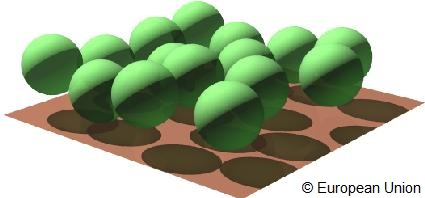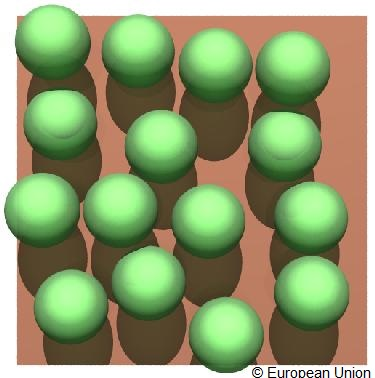RAMI website

# Turbid

RAMI3 phase

This set of experiments is the same as for RAMI phase 1 and 2 and requires to simulate the radiative transfer regime in the red and near infra-red spectral bands for heterogeneous environments. These scenes - which are structurally identical to those used for the turbid floating spheres test cases in the purist corner - are composed of a large number of non overlapping spherical objects representing the individual plant crowns, located over and only partially covering a horizontal plane standing for the underlying soil surface.

These objects, in turn, contained a 'gas' (to be treated as a turbid medium) of dimension-less particles representing the leaves. These scattering particles were characterized by specified radiative properties (reflectance, transmittance), and the orientation of the normals to the scatterers followed exclusively a uniform distribution function. The radiative properties of the underlying soil were also specified (in this case a simple Lambertian scattering law). The particular values selected for these input variables represented classical plant canopy conditions.

The following figures exhibit a graphical representation of such a scene, from the sideThe figures exhibit a graphical representation of such a scene, from the side.The figures exhibit a graphical representation of such a scene, from the top.

The architecture of this scene (HET02) is precisely characterized as follows:

Scene dimensions:

 ( X × Y × Z) 100.0 × 100.0 × 30.0 [m × m × m] (Xmin, Ymin, Zmin) −50.0, −50.0, 0.0 [m, m, m] (Xmin, Ymax, Zmin) −50.0, +50.0, 0.0 [m, m, m] (Xmax, Ymin, Zmin) +50.0, −50.0, 0.0 [m, m, m] (Xmax, Ymax, Zmin) +50.0, +50.0, 0.0 [m, m, m] (Xmin, Ymin, Zmax) −50.0, −50.0, 30.0 [m, m, m] (Xmin, Ymax, Zmax) −50.0, +50.0, 30.0 [m, m, m] (Xmax, Ymin, Zmax) +50.0, −50.0, 30.0 [m, m, m] (Xmax, Ymax, Zmax) +50.0, +50.0, 30.0 [m, m, m] Scatterer shape Disc of negligible thickness Scatterer radius 0 or close to 0 [m] LAI of individual sphere 5.0 Scatterer normal distribution Uniform Number of spheres 15 Fractional scene area coverage of spheres 0.471 Sphere radius 10.0 [m] Minimum sphere center height 11.0 [m] Maximum sphere center height 19.0 [m]

where the Leaf Area Index (LAI) is calculated as follows:

LAI = (# of leaves × one-sided area of single leaf) ⁄ (π × square of the radius of sphere)

An ASCII file with the radius (R), centre coordinates (Xc,Yc,Zc), and direction cosines (Dx,Dy,Dz) of every single leaf in a spherical volumes centered at 0,0,0 can be found here. This file (is ~ 2.2 Mbytes and) contains 49999 lines of format R Xc Yc Zc Dx Dy Dz and may serve as input to your scene creation process (provided that you are able to create multiple instances of its content, each one of which is then translated to the actual locations of the sphere centers in the scene. The coordinates (X, Y, Z) of the various sphere centers are as follows:

 −24.8302; 11.6110; 15.6210 −38.3380; −9.06342; 17.6094 −31.3562; 32.5297; 14.3051 11.9126; 8.32062; 12.1220 39.4855; 37.1247; 16.3647 −8.30999; −4.39422; 14.4241 12.2769; −25.1402; 12.4492 32.4756; −26.9023; 16.3684 −7.46991; −32.2138; 12.6678 −27.4794; −32.0266; 15.9146 33.5709; −6.31039; 14.5332 −5.24326; 39.8405; 16.8247 18.1699; 35.8223; 11.5683 −5.26569; 18.9618; 17.2535 37.8226; 14.6769; 11.5936

The underlying soil is occupying the entire lower surface of the scene, i.e., the area enclosed between the following coordinates: (−50.0, −50.0, 0.0), (−50.0, +50.0, 0.0), (+50.0, −50.0, 0.0), and (+50.0, +50.0, 0.0), where all values are in meters.

The tables below provide the details required to execute each of the experiments in this category. Every table is preceeded by the corresponding experiment identifier tag that is needed in the naming of the various measurement results files (see file naming and formatting conventions).

# brfop

Results for BRF in the cross plane (perpendicular to the principal plane)

# brfop_co_sgl

Results for BRF in the cross plane for single-scattered radiation

# brfop_mlt

Results for BRF in the cross plane for multiple-scattered (two or more scattering events) radiation

# brfop_uc_sgl

Results for BRF in the cross plane for single-scattered radiation collided by the soil

# brfpp

Results for BRF in the principal plane (total)

# brfpp_co_sgl

Results for BRF in the principal plane for single-scattered radiation

# brfpp_mlt

Results for BRF in the principal plane for multiple-scattered (two or more scattering events) radiation

# brfpp_uc_sgl

Results for BRF in the principal plane for single-scattered radiation collided by the soil

# fabs

Results for Absorption by the canopy (turbid or discrete)

# ftran

Results for Total transmission through the medium at the lower boundary level